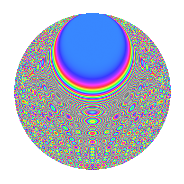# Properties

 Label 2304.2.yLevel $2304$ Weight $2$ Character orbit 2304.y Rep. character $\chi_{2304}(191,\cdot)$ Character field $\Q(\zeta_{12})$ Dimension $384$ Sturm bound $768$

# Related objects

## Defining parameters

 Level: $$N$$ $$=$$ $$2304 = 2^{8} \cdot 3^{2}$$ Weight: $$k$$ $$=$$ $$2$$ Character orbit: $$[\chi]$$ $$=$$ 2304.y (of order $$12$$ and degree $$4$$) Character conductor: $$\operatorname{cond}(\chi)$$ $$=$$ $$144$$ Character field: $$\Q(\zeta_{12})$$ Sturm bound: $$768$$

## Dimensions

The following table gives the dimensions of various subspaces of $$M_{2}(2304, [\chi])$$.

Total New Old
Modular forms 1632 384 1248
Cusp forms 1440 384 1056
Eisenstein series 192 0 192

## Trace form

 $$384q + O(q^{10})$$ $$384q - 192q^{49} + O(q^{100})$$

## Decomposition of $$S_{2}^{\mathrm{new}}(2304, [\chi])$$ into newform subspaces

The newforms in this space have not yet been added to the LMFDB.

## Decomposition of $$S_{2}^{\mathrm{old}}(2304, [\chi])$$ into lower level spaces

$$S_{2}^{\mathrm{old}}(2304, [\chi]) \cong$$ $$S_{2}^{\mathrm{new}}(144, [\chi])$$$$^{\oplus 5}$$$$\oplus$$$$S_{2}^{\mathrm{new}}(576, [\chi])$$$$^{\oplus 3}$$$$\oplus$$$$S_{2}^{\mathrm{new}}(1152, [\chi])$$$$^{\oplus 2}$$

## Hecke characteristic polynomials

There are no characteristic polynomials of Hecke operators in the database